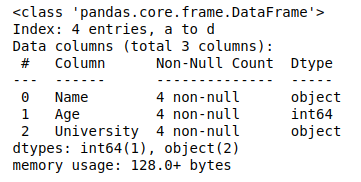Open in App
Not now

# Get the number of rows and number of columns in Pandas Dataframe

• Last Updated : 02 Jul, 2020

Pandas provide data analysts a variety of pre-defined functions to Get the number of rows and columns in a data frame. In this article, we will learn about the syntax and implementation of few such functions.

#### Method 1: Using df.axes() Method

`axes()` method in pandas allows to get the number of rows and columns in a go. It accepts the argument ‘0’ for rows and ‘1’ for columns.

Syntax: df.axes[0 or 1]

Parameters:
0: for number of Rows
1: for number of columns

Example:

 `# import pandas library``import` `pandas as pd``   ` `# dictionary with list object in values``details ``=` `{``    ``'Name'` `: [``'Ankit'``, ``'Aishwarya'``, ``'Shaurya'``, ``'Shivangi'``],``    ``'Age'` `: [``23``, ``21``, ``22``, ``21``],``    ``'University'` `: [``'BHU'``, ``'JNU'``, ``'DU'``, ``'BHU'``],``}``   ` `# creating a Dataframe object ``df ``=` `pd.DataFrame(details, columns ``=` `[``'Name'``, ``'Age'``, ``'University'``],\``                  ``index ``=` `[``'a'``, ``'b'``, ``'c'``, ``'d'``])``   ` `# Get the number of rows and columns``rows ``=` `len``(df.axes[``0``])``cols ``=` `len``(df.axes[``1``])`` ` `# Print the number of rows and columns``print``(``"Number of Rows: "` `+` `str``(rows))``print``(``"Number of Columns: "` `+` `str``(cols))`

Output:

```Number of Rows: 4
Number of Columns: 3
```

#### Method 2: Using df.info() Method

`df.info()` method provides all the information about the data frame, including the number of rows and columns.

Syntax:

`df.info`

Example:

 `# import pandas library``import` `pandas as pd``   ` `# dictionary with list object in values``details ``=` `{``    ``'Name'` `: [``'Ankit'``, ``'Aishwarya'``, ``'Shaurya'``, ``'Shivangi'``],``    ``'Age'` `: [``23``, ``21``, ``22``, ``21``],``    ``'University'` `: [``'BHU'``, ``'JNU'``, ``'DU'``, ``'BHU'``],``}``   ` `# creating a Dataframe object ``df ``=` `pd.DataFrame(details, columns ``=` `[``'Name'``, ``'Age'``, ``'University'``],``                  ``index ``=` `[``'a'``, ``'b'``, ``'c'``, ``'d'``])``   ` `# Get the info of data frame``df.info()`

Output:Here in the above code, the value in the Index gives the number of rows and the value in Data columns gives the number of columns.

#### Method 3: Using len() Method

`len()` method is used to get the number of rows and number of columns individually.

Syntax:

```len(df)
and
len(df.columns)```

Example 1: Get the number of rows

 `# import pandas library``import` `pandas as pd``   ` `# dictionary with list object in values``details ``=` `{``    ``'Name'` `: [``'Ankit'``, ``'Aishwarya'``, ``'Shaurya'``, ``'Shivangi'``],``    ``'Age'` `: [``23``, ``21``, ``22``, ``21``],``    ``'University'` `: [``'BHU'``, ``'JNU'``, ``'DU'``, ``'BHU'``],``}``   ` `# creating a Dataframe object ``df ``=` `pd.DataFrame(details, columns ``=` `[``'Name'``, ``'Age'``, ``'University'``],``                  ``index ``=` `[``'a'``, ``'b'``, ``'c'``, ``'d'``])``   ` `# Get the number of rows``print``(``"Number of Rows:"``, ``len``(df))`

Output:

```Number of Rows: 4
```

Example 2: Get the number of columns

 `# import pandas library``import` `pandas as pd``   ` `# dictionary with list object in values``details ``=` `{``    ``'Name'` `: [``'Ankit'``, ``'Aishwarya'``, ``'Shaurya'``, ``'Shivangi'``],``    ``'Age'` `: [``23``, ``21``, ``22``, ``21``],``    ``'University'` `: [``'BHU'``, ``'JNU'``, ``'DU'``, ``'BHU'``],``}``   ` `# creating a Dataframe object ``df ``=` `pd.DataFrame(details, columns ``=` `[``'Name'``, ``'Age'``, ``'University'``],``                  ``index ``=` `[``'a'``, ``'b'``, ``'c'``, ``'d'``])``   ` `# Get the number of columns``print``(``"Number of Columns:"``, ``len``(df.columns))`

Output:

```Number of Columns: 3
```

#### Method 4: Using df.shape() Method

`df.shape()` method returns the number of rows and columns in the form of a tuple.

Example:

 `# import pandas library``import` `pandas as pd``   ` `# dictionary with list object in values``details ``=` `{``    ``'Name'` `: [``'Ankit'``, ``'Aishwarya'``, ``'Shaurya'``, ``'Shivangi'``],``    ``'Age'` `: [``23``, ``21``, ``22``, ``21``],``    ``'University'` `: [``'BHU'``, ``'JNU'``, ``'DU'``, ``'BHU'``],``}``   ` `# creating a Dataframe object ``df ``=` `pd.DataFrame(details, columns ``=` `[``'Name'``, ``'Age'``, ``'University'``],``                  ``index ``=` `[``'a'``, ``'b'``, ``'c'``, ``'d'``])``   ` `# Get the number of Rows and columns``df.shape`

Output:

`(4, 3)`

My Personal Notes arrow_drop_up